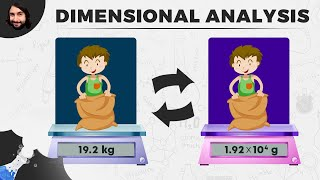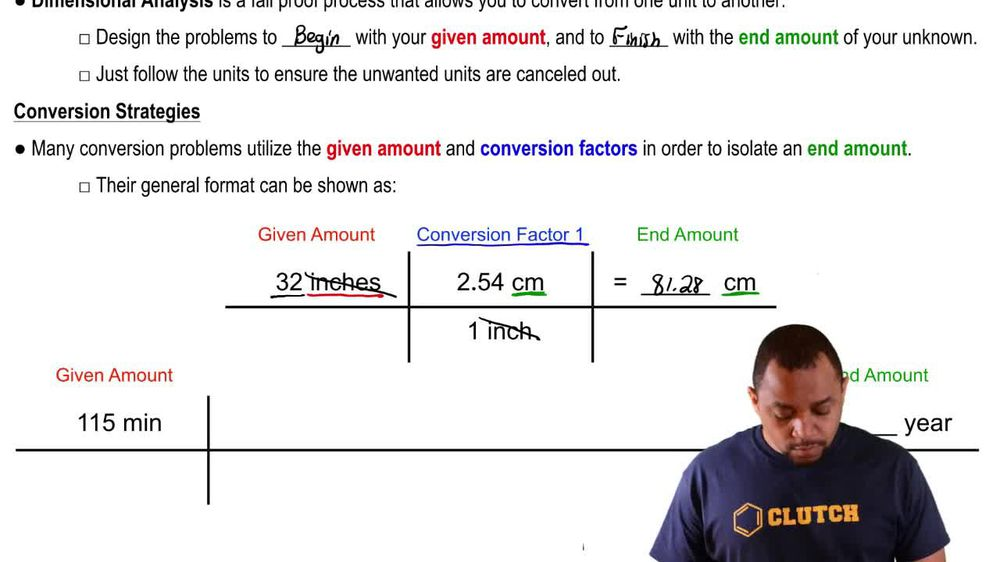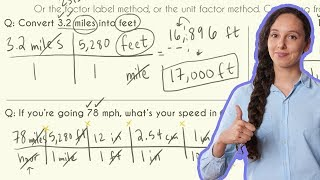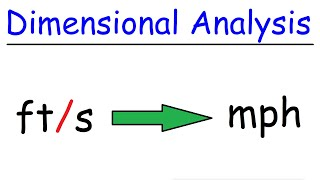Start typing, then use the up and down arrows to select an option from the list.
1. 1. Intro to General Chemistry2. Dimensional Analysis
Problem

# The average U.S. farm occupies 435 acres. How many square miles is this? (1 acre = 43,560 ft2, 1 mile = 5280 ft)

Relevant Solution2m
Play a video:
Hi everyone here we have a question telling us that the total United States land area is 3.12 million square miles and 539 million acres of it is covered by forests. Our goal is to calculate the forest area and miles squared and it's percentage relative to the total area. One acre equals 43,560 ft squared and one mile equals 5280 ft. So we have five Times 10 to the six acres Times 43, 560 ft squared, over one acre times one mile over ft squared. And our acres are canceling out. Our feet squared are canceling out and that equals 8.4, 2 Times 10 to the 5th mile squared. So our percentage relative to the total area is going to be 8.42 times 10 to the fifth miles squared, divided by 3.1, 2 times 10 to the 6th miles squared. And our Mild Square are going to cancel out. So this will be you nicolas. And then we're gonna be times that by 100 and that is going to equal .03%. And those are our two final answers. Thank you for watching. Bye